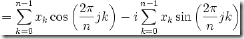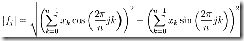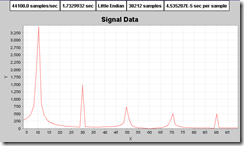## Tuesday, October 18, 2005

### Obtaining the Frequency Content of a Sampled Sound Using Java and the Discrete Fourier Transform

Sampled Sound as a Data Structure

In Java sampled sounds are stored as a series of bytes. A byte is a grouped series of 8 bits, where a bit is a binary number (either 1 or 0). This series is also stored as an array, where an array represents a series of values at specific indexes denoted by brackets. For example if array a[] contains all numbers 1 through 3 starting with 1, a = 1, a = 2, and a = 3. The AudioFormat object in Java encompasses all relevant data about a sound such as encoding technique, number of channels, sample rate, number of bits per channel, frame rate, frame size in bytes, and byte-order. The only encoding technique this paper is concerned with is pulse code modulation (PCM). The number of channels denotes whether a sampled sound is mono or stereo and the number of channels involved. The sample rate is the number of samples per second per channel. The number of bits per channel denotes how many binary numbers are stored per channel. The frame rate in PCM is equal to the sample rate, and the frame size in bytes is the number of channels multiplied by the sample size in bits, divided by the number of bits in a byte. The byte-order is the order in which bytes are stored.1

Byte-order can be Big Endian or Little Endian. Little Endian means that the low-order byte of the number is stored in memory at the lowest address, and the high-order byte at the highest address.2 For example the Little Endian 4-byte integer Byte3 Byte2 Byte1 Byte0 would be arranged in memory as Byte0 Byte1 Byte2 Byte3. Big Endian means that the high-order byte of the number is stored in memory at the lowest address, and the low-order byte at the highest address.3 For example the Big Endian 4-byte integer Byte3 Byte2 Byte1 Byte0 would be arranged in memory as Byte3 Byte2 Byte1 Byte0.

Basics of Java Sound Manipulation

```/* This code is based on the example found at:
http://www.jsresources.org/examples/ClipPlayer.java.html */```
```//Load the sound file
File soundFile = new File("sin300.wav");

//Object that will hold the audio input stream for the sound
Object currentSound;

//Object that will play the audio input stream of the sound
Clip clip;

//Object that contains format information about the audio input stream
AudioFormat format;

//Load the audio input stream
try {
currentSound = AudioSystem.getAudioInputStream(soundFile);
} catch(Exception e1) {
System.out.println(“Error loading file”);
}

try {
//Get the format information from the Audio Input Stream
AudioInputStream stream = (AudioInputStream) currentSound;
format = stream.getFormat();

//Get information about the line
DataLine.Info info = new DataLine.Info(Clip.class,
stream.getFormat(),
((int) stream.getFrameLength() * format.getFrameSize()));

//Load clip information from the line information
clip = (Clip) AudioSystem.getLine(info);

//Write the stream onto the clip
clip.open(stream);

//make the current sound the clip
currentSound = clip;

//start the clip
clip.start();

//loop the clip continuously
clip.loop(Clip.LOOP_CONTINUOUSLY);

} catch (Exception ex) {
ex.printStackTrace();
currentSound = null;
}```

4

This code plays a given sound file over and over again by looping through the byte array of audio data that can be obtained through the AudioFormat object, but this code by itself is of little use since no operations are performed on the sampled sound. In order to perform operations on the sampled sound the FloatControl object has to be used. The FloatControl object provides control over a range of floating-point values,5 which are the types of data found in a sampled sound. The most straightforward method for frequency manipulation of a sampled sound is changing the sample rate. The sample rate is the rate at which periodic measurements are taken of instantaneous amplitudes of an electric signal.6 Doubling the sample rate of a sampled sound causes the frequency to double and the duration to be halved. Halving the sample rate of a sampled sound causes the frequency to be halved and the duration to double. Manipulating the sample rate using the FloatControl object can be done in the following way:

```//Get the sampling rate from the AudioFormat object in the code above and double it
float newRate = format.getSampleRate() * 2;

//Create the FloatControl object with the clip object from the code above
FloatControl rateControl = (FloatControl) clip.getControl(FloatControl.Type.SAMPLE_RATE);

//change the sampling rate
rateControl.setValue(newRate);```

7

If the Clip and AudioFormat objects are made global, this code can be called as a thread while the sampled sound is playing to change its frequency in real-time. The other sampled sound manipulation needed is the ability to change sound level. Sound level manipulation can also be done through using the FloatControl object:

```//specify a new sound level from 0 to 100
double gain = 80.0;

//create the gain control object using the clip object from the code above
FloatControl control = (FloatControl) clip.getControl(FloatControl.Type.MASTER_GAIN);

//convert the given sound level to decibel
gain = gain / 100;
float dB = (float) (Math.log(gain == 0.0 ? 0.0001 :gain) / Math.log(10.0)*20.0);

//change the sound level
control.setValue(dB);```

8

Sample Rate Manipulation Issues

Changing the frequency of a sampled sound by modifying the sample rate can cause some potential problems though, especially when dealing with sounds that have complex harmonic spectrums. A single sound such as that of musical instrument contains a multitude of additional frequencies that make up its harmonic spectrum. The problem is that a sampled sound system can only handle sound data where the maximum frequency content is half that of the sample rate, which is called the Nyquist limit. Frequencies above the Nyquist limit create unwanted signals in the band of interest creating an effect called aliasing.9 An example of aliasing can be seen in almost any car commercial if you stare at the wheels of a car that is moving at a constant velocity. You will notice that the wheels rotate forward for a short time, slow down, and then appear to move backwards. This effect is the result of the frequency of wheel rotation being higher than half that of the frame rate (sampling rate) of the camera.

The solution to the aliasing problem is to use an anti-aliasing filter. Anti-aliasing works by using the Fast Fourier Transform (FFT) to break a digital signal into its basic waves (harmonics) and their corresponding amplitudes, and blocking out those harmonics that are at frequencies above the Nyquist limit.10

The Discrete Fourier Transform

Fourier transforms are used to convert digital signals from the time domain into the frequency domain by converting the signal information to a magnitude and phase component of each frequency.11 Magnitude describes a number assigned to the ratio of two quantities,12 while phase describes a measure of relative time between corresponding points on two identical waves.13 For my purposes though I am only interested in the amplitudes of all harmonics within a sound, so I can use a Discrete Fourier Transform (DFT). Signal analysis using DFT works in the following way:

Let x(t) represent the function of a digital signal
Where t is a time interval ranging [0,T], meaning that t can be any value from 0 to T
The digital signal represented by the function x(t) is sampled at n equidistant points in time
The function x(t) will be calculated for each time interval up to the sampling rate – 1
With values of t being represented by h where h = T/ n
For x0 = x(0), x1 = x(h), x2 = x(2h), … , xn-1 = x( (n-1)h )
A DFT, represented by fj, is then calculated for each value of xj

14

By using Euler’s formula on the DFT equation:151617

The DFT equation can be simplified to:18

The DFT Algorithm
Before expressing DFT as an algorithm data storage and representation must be handled. Also the digital signal first must be stored in an array of bytes. Getting a sound file in this format is similar to what was done in the previous example, with a few major changes:

```/* This code is based on the example found at:
http://www.jsresources.org/examples/SimpleAudioPlayer.java.html */```
```//Create a global buffer size
private static final int EXTERNAL_BUFFER_SIZE = 128000;

//Get the location of the sound file
File soundFile = new File("sin300.wav");

//Load the Audio Input Stream from the file
AudioInputStream audioInputStream = null;
try {
audioInputStream = AudioSystem.getAudioInputStream(soundFile);
} catch (Exception e) {
e.printStackTrace();
System.exit(1);
}

//Get Audio Format information
AudioFormat audioFormat = audioInputStream.getFormat();

//Handle opening the line
SourceDataLine	line = null;
DataLine.Info	info = new DataLine.Info(SourceDataLine.class, audioFormat);
try {
line = (SourceDataLine) AudioSystem.getLine(info);
line.open(audioFormat);
} catch (LineUnavailableException e) {
e.printStackTrace();
System.exit(1);
} catch (Exception e) {
e.printStackTrace();
System.exit(1);
}

//Start playing the sound
line.start();

//Write the sound to an array of bytes
int nBytesRead = 0;
byte[]	abData = new byte[EXTERNAL_BUFFER_SIZE];
while (nBytesRead != -1) {
try {
nBytesRead = audioInputStream.read(abData, 0, abData.length);

} catch (IOException e) {
e.printStackTrace();
}
if (nBytesRead >= 0) {
int nBytesWritten = line.write(abData, 0, nBytesRead);
}

}

//close the line
line.drain();
line.close();```

19

With the sound objects such as AudioFormat and AudioInputStream initialized, some of the variables needed for DFT computations can be calculated:

```//Calculate the sample rate
float sample_rate = audioFormat.getSampleRate();
System.out.println("sample rate = "+sample_rate);

//Calculate the length in seconds of the sample
float T = audioInputStream.getFrameLength() / audioFormat.getFrameRate();
System.out.println("T = "+T+ " (length of sampled sound in seconds)");

//Calculate the number of equidistant points in time
int n = (int) (T * sample_rate) / 2;
System.out.println("n = "+n+" (number of equidistant points)");

//Calculate the time interval at each equidistant point
float h = (T / n);
System.out.println("h = "+h+" (length of each time interval in seconds)");```

20

When this code is run using the example sampled sound (sqr-10Hz.wav) it prints out the DFT variables calculated so far:

```sample rate = 44100.0
T = 1.7329932 (length of sampled sound in seconds)
n = 38212 (number of equidistant points)
h = 4.535207E-5 (length of each time interval in seconds)```

This array of bytes though does not directly contain signal data per time interval, which is needed in order to perform DFT. The array of bytes must be converted to Endian format where the specific type of Endian is dependant on the original encoding of the sampled sound. Each value in the byte array is 8 bits or 1 byte, and Endian values are stored in the format of 16 bits or 2 bytes, meaning that it takes 2 byte values from the byte array to represent the signal data at a given point in time, where the signal data at a given point in time is a single Endian value. The conversion can be done in the following way:

```//Determine the original Endian encoding format
boolean isBigEndian = audioFormat.isBigEndian();

//this array is the value of the signal at time i*h
int x[] = new int[n];

//convert each pair of byte values from the byte array to an Endian value
for (int i = 0; i < n*2; i+=2) {
int b1 = abData[i];
int b2 = abData[i + 1];
if (b1 < 0) b1 += 0x100;
if (b2 < 0) b2 += 0x100;

int value;

//Store the data based on the original Endian encoding format
if (!isBigEndian) value = (b1 << 8) + b2;
else value = b1 + (b2 << 8);
x[i/2] = value;
}```

21

The array x now contains the signal values at each time interval, so the DFT can be performed on each value of x.

It should also be noted that only the first half of the equidistant points are useable due to the Nyquist Criterion, because data will only be reliable for half of the sampling frequency (sample rate). The frequency is also a combination of placement along the time domain, sample rate, the number of equidistant points, and the length of each equidistant point in seconds.

```//do the DFT for each value of x sub j and store as f sub j
double f[] = new double[n/2];
for (int j = 0; j < n/2; j++) {

double firstSummation = 0;
double secondSummation = 0;

for (int k = 0; k < n; k++) {
double twoPInjk = ((2 * Math.PI) / n) * (j * k);
firstSummation +=  x[k] * Math.cos(twoPInjk);
secondSummation += x[k] * Math.sin(twoPInjk);
}

f[j] = Math.abs( Math.sqrt(Math.pow(firstSummation,2) +
Math.pow(secondSummation,2)) );

double amplitude = 2 * f[j]/n;
double frequency = j * h / T * sample_rate;
System.out.println("frequency = "+frequency+", amp = "+amplitude);
}```

22

Testing the DFT Algorithm

Given a sampled sound in which the frequency is known (sqr-10Hz.wav), a sound containing a 10 Hz square wave, the validity of this process can be tested.

Because this process generated a lot of data, it is easier to view the result as a plot instead of a 38,212 line long list of frequencies and amplitudes. I used a free plotting package called JFreeChart (www.jfree.org) to plot the resulting data.

Time Domain:

Frequency Domain:

Frequency Domain Zoomed In: